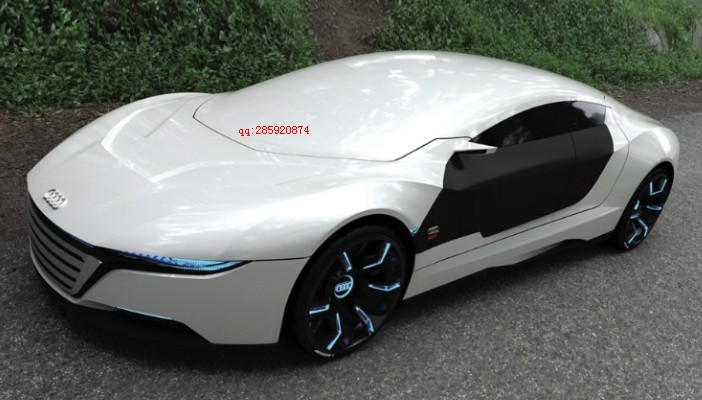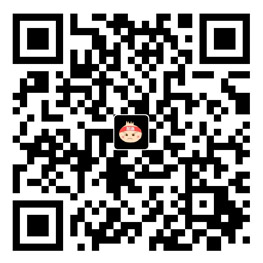# 网友C#2010开发UG7.0心得3（旋转坐标系与球）

C#2010开发UG7.0心得3（旋转坐标系与球）

workPart.WCS.Rotate(NXOpen.WCS.Axis.ZAxis,60.0);BlkFeatureBuilder.SetOriginAndLengths(origin, Length, Width, Height);

afterX = originalX * System.Math.Cos(化弧度(60)) + originalY * System.Math.Sin(化弧度(60));
afterY = originalX * System.Math.Sin(化弧度(60)) – originalY * System.Math.Cos(化弧度(60));

public double 化弧度(double Degree)
{
return Degree * System.Math.PI / 180.0;
}

public void ResumeWCS()
{
Point3d origin = new Point3d(0.0, 0.0, 0.0);
Matrix3x3 matrix;
matrix.Xx = 1.0;
matrix.Xy = 0.0;
matrix.Xz = 0.0;
matrix.Yx = 0.0;
matrix.Yy = 1.0;
matrix.Yz = 0.0;
matrix.Zx = 0.0;
matrix.Zy = 0.0;
matrix.Zz = 1.0;
workPart.WCS.SetOriginAndMatrix(origin, matrix);
}

NXOpen.Features.Sphere nullFeatures_Sphere = null;
NXOpen.Features.SphereBuilder sphereBuilder1;
sphereBuilder1 = workPart.Features.CreateSphereBuilder(nullFeatures_Sphere);

sphereBuilder1.Diameter.RightHandSide = “20”;
Point3d basepoint = new Point3d(10.0, 10.0, 10.0);
Point point1= workPart.Points.CreatePoint(basepoint);
sphereBuilder1.CenterPoint = point1;

NXObject nXObject1;
nXObject1 = sphereBuilder1.Commit();
sphereBuilder1.Destroy();

### 觉得文章有用就打赏一下文章作者

#### 支付宝扫一扫打赏#### 微信扫一扫打赏